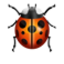## Welcome to the Treehouse Community

The Treehouse Community is a meeting place for developers, designers, and programmers of all backgrounds and skill levels to get support. Collaborate here on code errors or bugs that you need feedback on, or asking for an extra set of eyes on your latest project. Join thousands of Treehouse students and alumni in the community today. (Note: Only Treehouse students can comment or ask questions, but non-students are welcome to browse our conversations.)

### Looking to learn something new?

Treehouse offers a seven day free trial for new students. Get access to thousands of hours of content and a supportive community. Start your free trial today.

# LINQ Method Syntax challenge

In the preceding video the LINQ query

```from b in birds
where b.Color == "Red"
select b;
```

is refactored in 3 different ways:

```birds.Where((b) => b.Color == "Red");
birds.Where((b) => { return b.Color == "Red"; });
birds.Where(b => b.Color == "Red");
```

I've tried all 3 variants in the challenge and for each I get the error message: Bummer! Did you use

And that's it. Just Did you use

Did I use what?

I'm currently out of thoughts? If anyone has a suggestion it would be welcome! Thanks.

NumberAnalysis.cs
```using System.Collections.Generic;
using System.Linq;

namespace Treehouse.CodeChallenges
{
public class NumberAnalysis
{
private List<int> _numbers;
public NumberAnalysis()
{
_numbers = new List<int> { 2, 4, 6, 8, 10 };
}

public IEnumerable<int> NumbersGreaterThanFive()
{
//return from n in _numbers where n > 5 select n;  //LINQ query

//return _numbers.Where((n) => n > 5);  //version 1
//return _numbers.Where((n) => { return n > 5; });  //version 2a
//_numbers.Where((n) => { return n > 5; });  //version 2b
return _numbers.Where(n => n > 5); //version 3

}
}
}
```Congratulations, you've discovered a bug ("Did you use").You might want to report this to Treehouse Support.